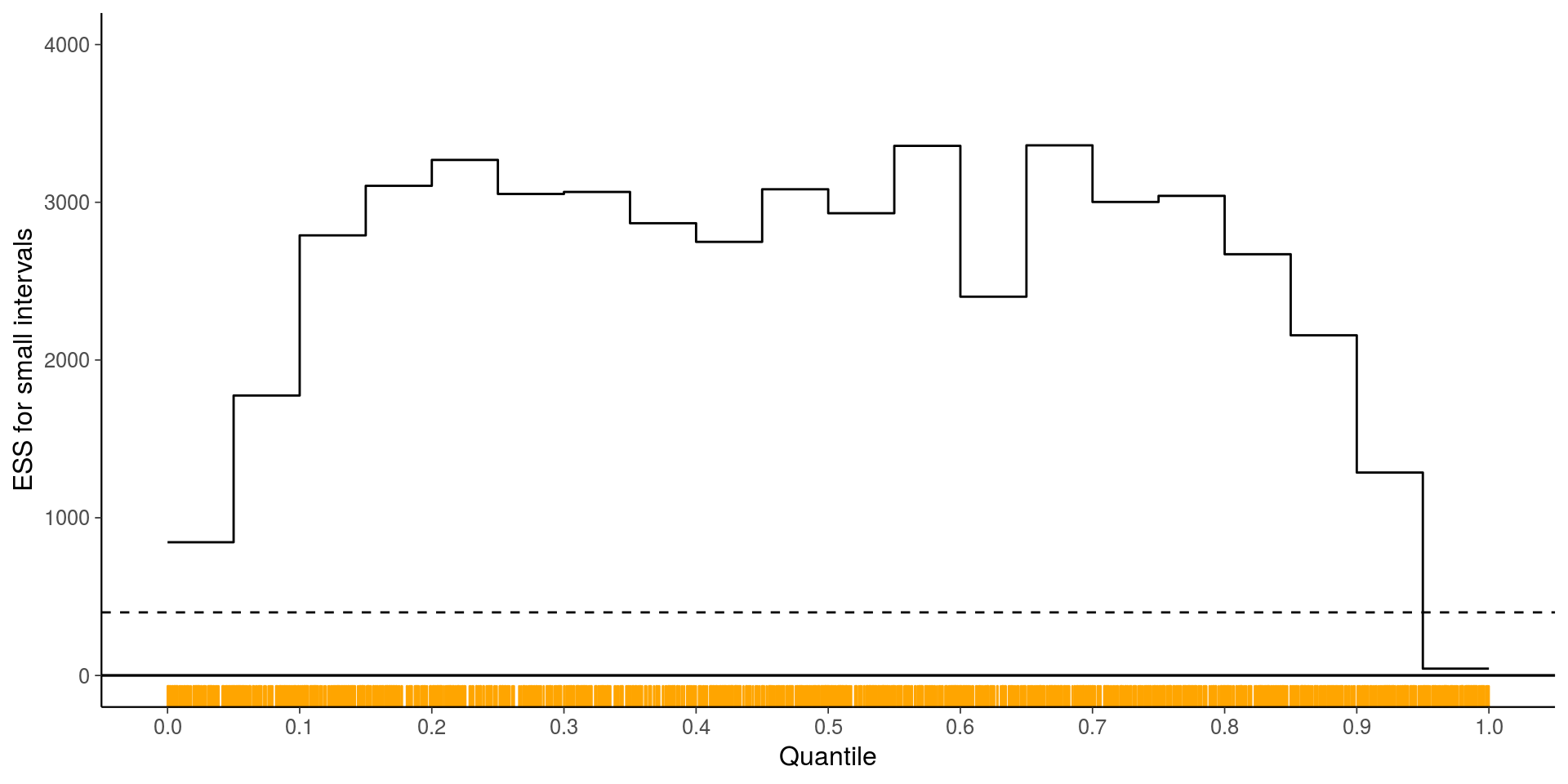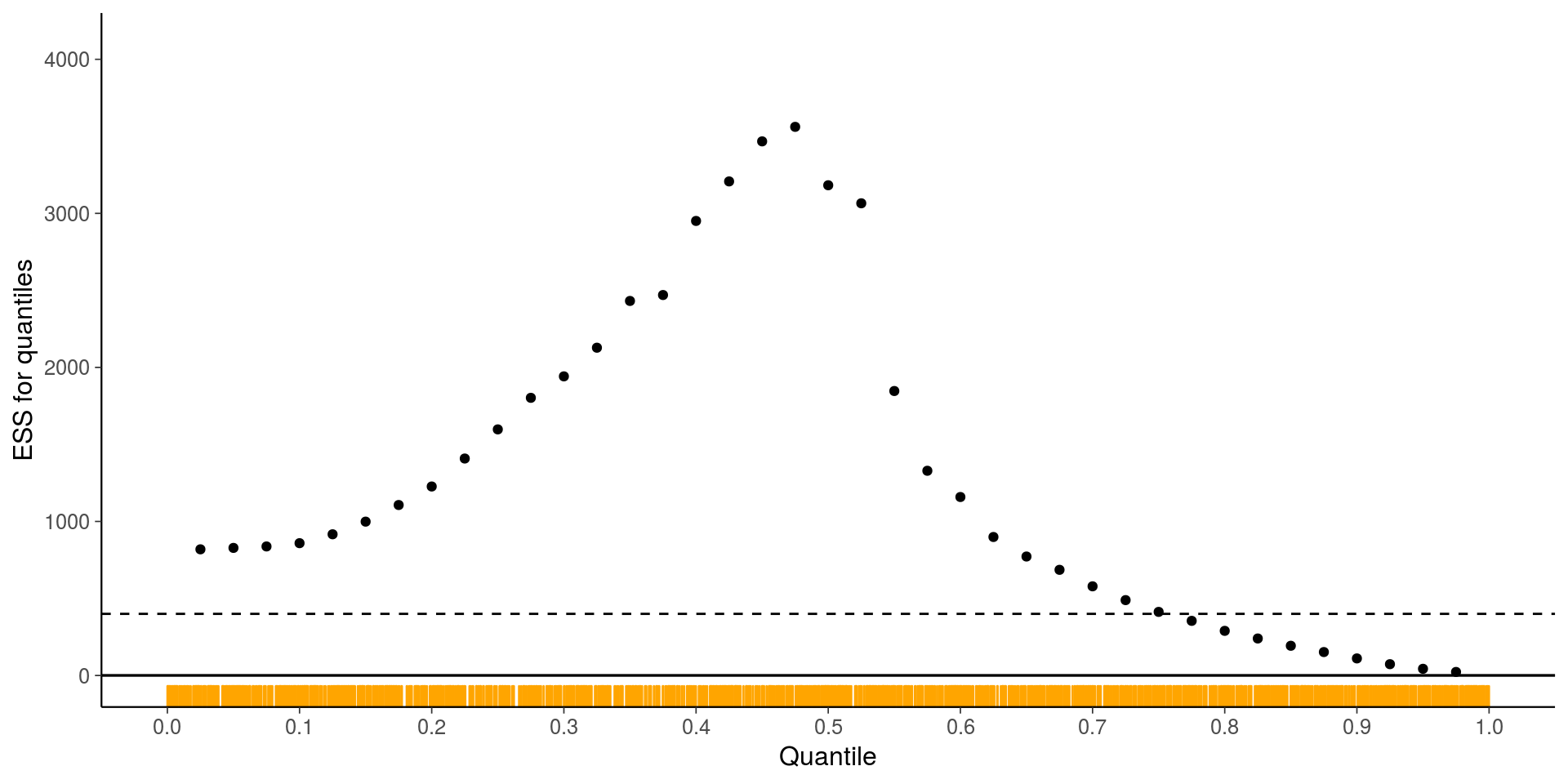# 1 Overview

This is the online appendix of the paper

• Aki Vehtari, Andrew Gelman, Daniel Simpson, Bob Carpenter, Paul-Christian Bürkner (2019): Rank-normalization, folding, and localization: An improved \(\widehat{R}\) for assessing convergence of MCMC. arXiv preprint arXiv:1903.08008.

The code for the paper is available on Github (https://github.com/avehtari/rhat_ess). Here, we introduce all the code related to the examples presented in the paper and more numerical experiments not discussed in the paper itself.

To help you finding your way through all the examples presented in this online appendix, below please find a list of links to the examples discussed in the paper:

# 2 Examples

In this section, we will go through some examples to demonstrate the usefulness of our proposed methods as well as the associated workflow in determining convergence. Appendices B-E contain more detailed analysis of different algorithm variants and further examples.

``````library(tidyverse)
library(gridExtra)
library(rstan)
options(mc.cores = parallel::detectCores())
rstan_options(auto_write = TRUE)
library(bayesplot)
theme_set(bayesplot::theme_default(base_family = "sans"))
library(rjags)
library(abind)
source('monitornew.R')
source('monitorplot.R')``````

## 2.1 Cauchy: A distribution with infinite mean and variance

This section relates to the examples presented in Section 5.1 of the paper.

Traditional \(\widehat{R}\) is based on calculating within and between chain variances. If the marginal distribution of a chain is such that the variance is infinite, this approach is not well justified, as we demonstrate here with a Cauchy-distributed example. The following Cauchy models are from Michael Betancourt’s case study Fitting The Cauchy Distribution. Appendix C contains more detailed analysis of different algorithm variants and further Cauchy examples.

### 2.1.1 Nominal parameterization of Cauchy

The nominal Cauchy model with direct parameterization is as follows:

``writeLines(readLines("cauchy_nom.stan"))``
``````parameters {
vector x;
}

model {
x ~ cauchy(0, 1);
}

generated quantities {
real I = fabs(x) < 1 ? 1 : 0;
}``````

#### 2.1.1.1 Default Stan options

Run the nominal model:

``fit_nom <- stan(file = 'cauchy_nom.stan', seed = 7878, refresh = 0)``
``````Warning: There were 1421 transitions after warmup that exceeded the maximum treedepth. Increase max_treedepth above 10. See
http://mc-stan.org/misc/warnings.html#maximum-treedepth-exceeded``````
``Warning: Examine the pairs() plot to diagnose sampling problems``

We get HMC specific diagnostic (Betancourt, 2017) warnings about a very large number of transitions after warmup that exceed the maximum treedepth and low estimated Bayesian fraction of missing information, indicating slow mixing likely due to very long tails of the Cauchy distribution.

``````mon <- monitor(fit_nom)
print(mon)``````
``````Inference for the input samples (4 chains: each with iter = 2000; warmup = 1000):

Q5    Q50    Q95   Mean    SD  Rhat Bulk_ESS Tail_ESS
x   -5.87   0.02   6.78   0.92 13.64  1.01     1967      360
x   -5.76  -0.03   5.11  -0.27  7.53  1.00     2417      802
x   -6.12   0.00   7.73   0.77 10.28  1.01      448       82
x   -5.30  -0.01   5.41  -0.06  7.19  1.01     2266      697
x  -13.14  -0.04   5.83  -2.78 17.58  1.03      169       45
x   -8.10  -0.06   6.39  -0.92 15.59  1.01     1611      402
x   -7.25  -0.08   7.55  -0.29  9.68  1.01     1227      462
x   -4.79   0.02   6.23   0.57  8.65  1.02     1568      432
x   -4.69   0.01   4.82   0.13  5.43  1.00     2607      784
x  -8.43  -0.04   6.50  -1.92 32.96  1.01     1609      261
x  -6.21   0.02   7.09   0.43 16.21  1.01     2464      474
x  -5.55   0.02   6.62   0.17  6.37  1.00     2813      404
x  -6.16   0.00   6.13   0.44 12.78  1.01     2282      565
x  -5.45   0.02   6.79   0.29  8.44  1.01     3121      647
x  -8.17   0.05   9.55   0.26 30.61  1.01     2126      521
x  -5.83   0.00   6.23   0.31  8.60  1.01     2177      589
x -18.10  -0.07   7.39  -6.89 47.38  1.03      209       67
x  -5.89   0.05   5.90   0.10  5.42  1.01     3336      664
x  -7.04   0.03   6.23  -0.23 12.26  1.01     1434      421
x  -6.58   0.03   7.53  -0.19  7.81  1.02      952      446
x  -8.11   0.01   6.57  -2.16 28.47  1.00     1790      397
x  -5.47   0.04   5.24   0.25  8.30  1.00     2841      712
x -10.04  -0.05   6.55 -11.16 87.97  1.04      318       47
x -13.18  -0.07   5.72  -4.58 30.89  1.01      441       98
x  -6.89  -0.02   5.60  -0.44  8.76  1.02     1892      490
x  -5.33  -0.02   4.69  -0.30  6.55  1.01     3099      732
x  -5.19  -0.04   5.17  -0.26  8.50  1.00     3096      874
x  -6.81  -0.02   6.81   0.15  7.71  1.00     4185      924
x  -9.96  -0.02   6.99  -1.66 18.87  1.01      935      223
x  -6.20   0.02   6.62   0.44 14.44  1.01     3504      559
x -18.84  -0.04   5.77  -4.22 29.48  1.02      210       49
x  -6.33  -0.01   6.21  -0.02  6.73  1.02     3039      748
x  -4.81   0.03   4.93   0.22  7.89  1.01     3505     1008
x  -5.75   0.02   5.75  -0.12  9.23  1.00     1896      661
x  -5.98   0.03   7.16   0.06  8.68  1.01     2706      639
x  -7.37   0.00   9.19   0.08 10.82  1.01     1405      399
x  -5.03   0.02   7.82   2.52 29.21  1.01     2029      357
x  -5.40  -0.01   5.59  -0.06  6.64  1.01     3611      741
x  -4.78  -0.02   5.36   0.27  6.40  1.00     2855      757
x  -6.16   0.00   4.58  -0.91 10.45  1.01     1860      457
x  -5.46   0.07   6.55   0.05  6.06  1.01     2328      620
x  -7.63  -0.05   6.38  -0.75 10.10  1.01     1796      513
x  -7.47  -0.04   6.39  -0.59  8.32  1.01     1876      558
x  -5.22   0.00   5.17   0.11  6.15  1.01     1610      575
x  -4.44   0.04  13.98   3.19 17.67  1.01      290       64
x  -5.04   0.01   5.60   0.22  9.06  1.02     3154      398
x  -5.87   0.03   5.46   0.19 11.73  1.02     2258      497
x  -4.99  -0.03   4.91   0.05 10.22  1.01     2916      724
x  -6.42   0.01  10.78  10.69 83.66  1.02      214       43
x  -6.24   0.02   5.71   0.41 19.28  1.00     2615      788
I       0.00   1.00   1.00   0.51  0.50  1.00      688      688
lp__  -88.54 -68.47 -50.77 -69.10 11.75  1.01      252      453

For each parameter, Bulk_ESS and Tail_ESS are crude measures of
effective sample size for bulk and tail quantities respectively (an ESS > 100
per chain is considered good), and Rhat is the potential scale reduction
factor on rank normalized split chains (at convergence, Rhat <= 1.01).``````
``which_min_ess <- which.min(mon[1:50, 'Tail_ESS'])``

Several values of Rhat greater than 1.01 and some ESS less than 400 also indicate convergence propblems. The Appendix C contains more results with longer chains.

We can further analyze potential problems using local efficiency and rank plots. We specifically investigate x, which, in this specific run, had the smallest tail-ESS of 43.

We examine the sampling efficiency in different parts of the posterior by computing the efficiency of small interval probability estimates (see Section Efficiency estimate for small interval probability estimates). Each interval contains \(1/k\) of the draws (e.g., \(5\%\) each, if \(k=20\)). The small interval efficiency measures mixing of an function which indicates when the values are inside or outside the specific small interval. As detailed above, this gives us a local efficiency measure which does not depend on the shape of the distribution.

``plot_local_ess(fit = fit_nom, par = which_min_ess, nalpha = 20)``The efficiency of sampling is low in the tails, which is clearly caused by slow mixing in long tails of the Cauchy distribution.
Orange ticks show iterations that exceeded the maximum treedepth.

An alternative way to examine the efficiency in different parts of the posterior is to compute efficiency estimates for quantiles (see Section Efficiency for quantiles). Each interval has a specified proportion of draws, and the efficiency measures mixing of a function which indicates when the values are smaller than or equal to the corresponding quantile.

``plot_quantile_ess(fit = fit_nom, par = which_min_ess, nalpha = 40)``Similar as above, we see that the efficiency of sampling is low in the tails. Orange ticks show iterations that exceeded the maximum treedepth.

We may also investigate how the estimated effective sample sizes change when we use more and more draws; Brooks and Gelman (1998) proposed to use similar graph for \(\widehat{R}\). If the effective sample size is highly unstable, does not increase proportionally with more draws, or even decreases, this indicates that simply running longer chains will likely not solve the convergence issues. In the plot below, we see how unstable both bulk-ESS and tail-ESS are for this example.

``plot_change_ess(fit = fit_nom, par = which_min_ess)``